How to use MIN function in Microsoft Excel

In this article, we will learn how to use MIN function in Microsoft Excel.

In Microsoft Excel, MIN function returns the smallest or minimum value from the provided numbers, named range or array.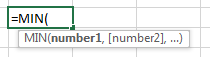Let’s take examples and understand:-

Here, we have a table with 5 different examples.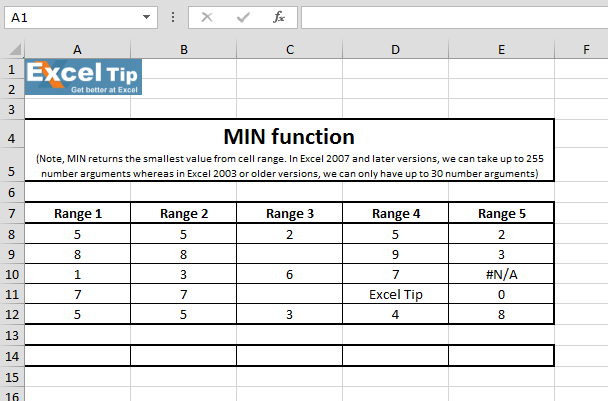1st Example:-

In this example, we have a range of numbers from which we want to return minimum value.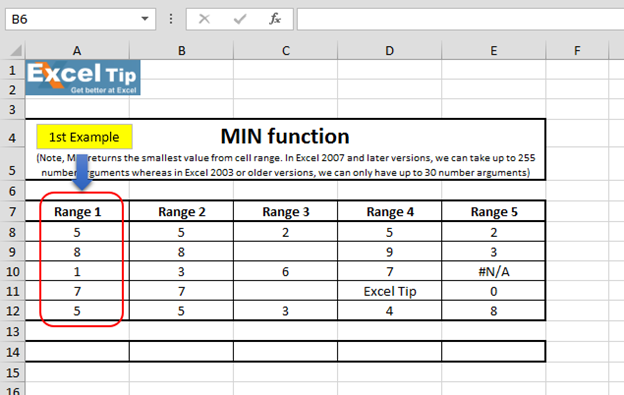• Enter the function in cell A14
• =MIN(A8:A12)
• Press Enter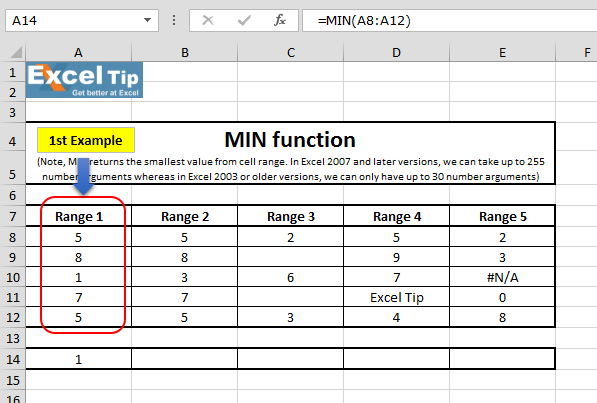• The function will return 1, which is the minimum value within the range

2nd Example:-

In this example, we will see what happens when we take any range in the 1st argument and enter any value in the 2nd argument.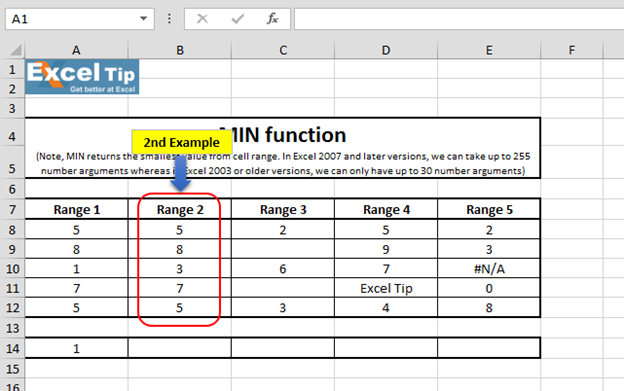• Enter the function in cell B14
• =MIN(B8:B12,2,3-2)
• Press Enter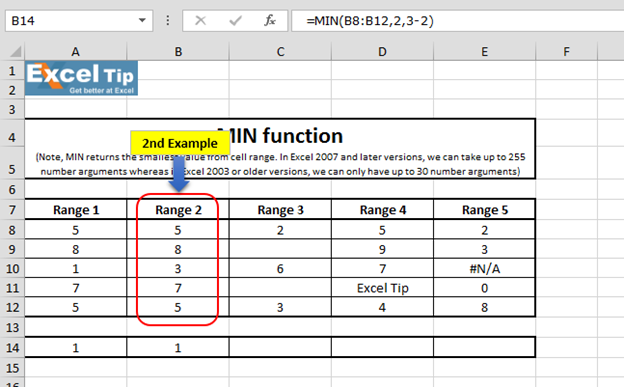Formula Explanation:-

In this formula, first we have selected the range from B8:B12 and then we have entered 3-2 which is equivalent to 1, so our minimum value is 1.

3rd Example:-

In this example, we will see what happens when we have blank cells within the range.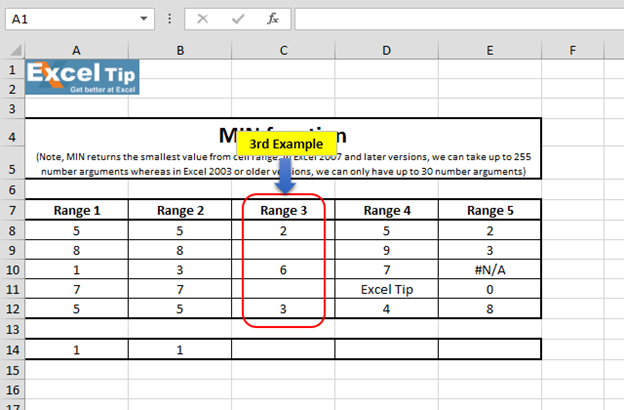• Enter the function in cell C14
• =MIN(C8:C12)
• Press Enter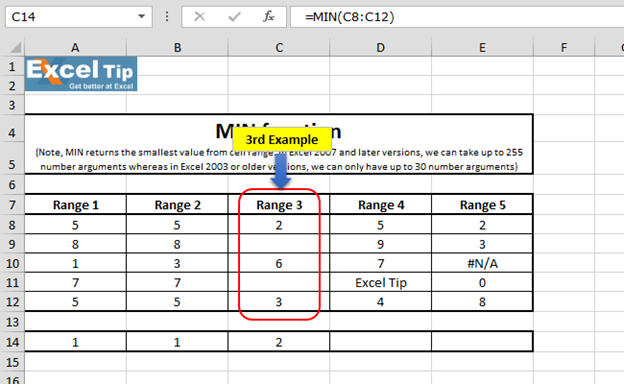Function has returned 2 as the minimum value. Therefore, we can say that function will look for the minimum value in available values only.

4th Example:-

In this example, we will see what happens when we have text value in the range for which we want to return minimum value.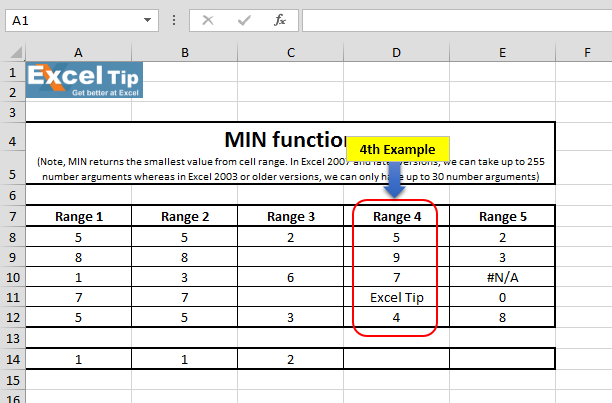• Enter the function in cell D14
• =MIN(D8:D12)
• Press Enter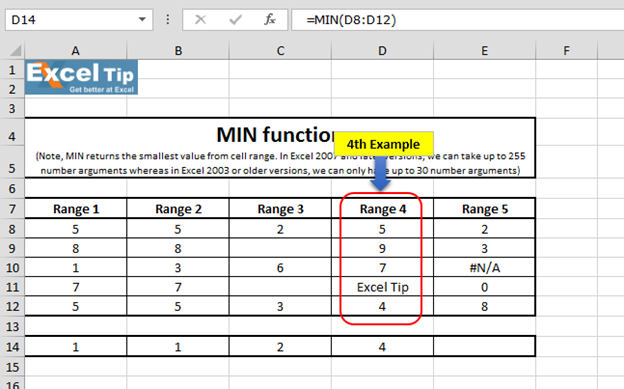The function has returned 4. This means while calculating, MIN function ignores text values as well.

5th Example:-

In this example, we have taken error value in the range for which we are going to calculate minimum value.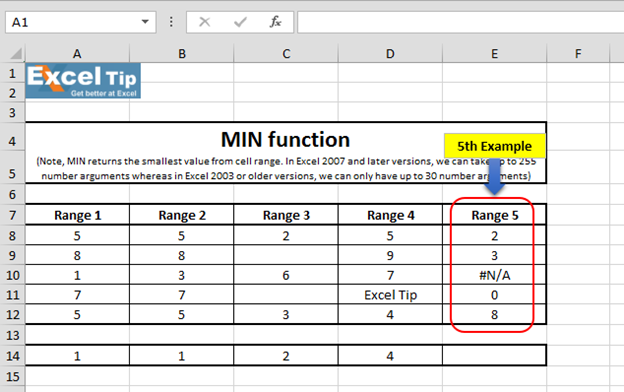• Enter the function in cell E14
• =MIN(E8:E12)
• Press Enter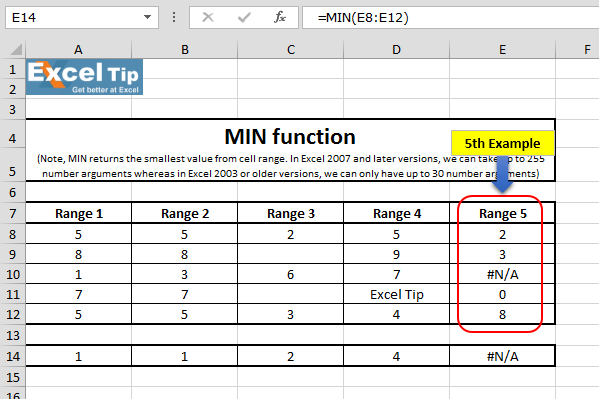It is because it cannot have any error value in the range which we are referring to in the number argument and hence it returns an error as an answer.

So, this is how MIN function works and we can make use of it in different situations.# Video: How to use MIN function in Microsoft Excel

Watch the steps in this short video, and the written instructions are above the video